# Intersubject Correlation¶

Written by Juha Lahnakoski and Luke Chang

Synchrony between individuals happens at several levels from behavior to brain activity (Nummenmaa et al., 2018, Nastase et al., 2019). To an observer, synchrony during interaction or joint motion (Hale et al., 2020) can reflect prosocial qualities such rapport (Miles et al., 2009) or affiliation (Hove and Risen, 2009). During physically arousing ritualistic experiences, observers may selectively synchronize their heart rates while observing related, but not unrelated individuals participating in the ritual (Konvalinka et al., 2011) and the degree of affective synchrony can predict the perceived social connection between two individuals (Cheong et al., 2020). Synchrony of brain activity is associated with, among other things, shared psychological perspectives toward a stimulus (Lahnakoski et al., 2014, Yeshurun et al., 2017, Yeshurun et al., 2017, Kang & Wheatley, 2017, Cheong et al., 2020) and friendship (Parkinson et al., 2018), and may also be disturbed in psychiatric conditions ranging from developmental conditions such as autism (Hasson et al., 2009; Salmi et al., 2013, Byrge et al., 2015) to more acute conditions such as first-episode psychosis (Mäntylä et al., 2018). Thus, measures of synchrony can offer a simple window to many psychological processes.

In brain imaging, synchrony of brain activity is most commonly measured using intersubject correlations (ISC; Hasson et al., 2004). As the name implies, this method calculates linear correlations between participants and derives summary statistics from these correlations to measure the level of similarity of brain activity. Overall, the brain activity measured with fMRI during naturalistic stimulation conditions can be thought to consist of four main sources: (1) stimulus-driven brain activity between individuals shared by all or most of the participants, (2) individual or idiosyncratic activity elicited by the stimulus, (3) intrinsic activity that is not time-locked to the stimulus, and (4) noise from various sources. The idea behind ISC is to identify brain activity that is shared by a large proportion of the participants (category 1). Thus, this method evaluates how much of an individual’s brain activity is explained by this shared component. By contrast, if smaller groups of participants, e.g. pairs of friends within the study, share similar individual activity patterns (category 2), it may be better captured by the dyadic values in the pairwise matrices using techniques such as IS-RSA (Parkinson et al., 2018, Chen et al., 2020, Finn et al., 2020). Generally, the third category of activity is not readily detected by synchrony approaches (Chang et al., 2018). However, with inventive experimental designs, e.g. during verbal recall of previously experienced stimuli (Chen et al., 2017), it is still possible to extract shared brain activity patterns by temporally reorganizing the data, even when the original experiences of the participants were out of sync. The optimal choice of analysis will depend on the research question and the type of shared activity patterns that are of particular interest. Most commonly, ISCs are calculated locally within each voxel (or region), but this approach has also been extended to functional connectivity (Simony et al., 2016). Intersubject correlations give a summary statistic of synchrony over long periods of time, usually over an entire imaging session. However, the level of synchrony may change considerably from one moment to the next with the experimental condition and can be investigated using time-varying measures of synchrony.

In this tutorial, we will cover:

• how to compute pairwise ISC

• how to perform hypothesis tests with ISC

• how to perform ISFC (also see the Dynamic Connectivity tutorial)

• how to perform dynamic ISC

Let’s get started by watching some short videos illustrating how ISC can be used with naturalistic data.

Dr. Yaara Yeshurun, PhD, an Assistant Professor at Tel-Aviv University, will provide two example applications of how ISC can be used to characterize the brain mechanisms underlying shared understanding.

from IPython.display import YouTubeVideo


YouTubeVideo('wEKKTG7Q1DQ')


## Computing Intersubject Correlation¶

### Pairwise vs Average Response¶

Generally, ISCs are calculated using one of two main approaches. First, one calculates pairwise correlations between all participant pairs to build a full intersubject correlation matrix. The second approach, uses the average activity timecourse of the other participants as a model for each individual left out participant. This procedure produces individual, rather than pairwise, spatial maps of similarity reflecting how similarly, or “typically”, each person’s brain activates. These maps lend themselves to similar analyses as one might perform with first level results of a traditional general linear model (GLM) analysis. However, some of the individual variability is lost with the latter approach, and thus the ISC values are typically of much higher magnitude compared to the pairwise matrices.

### Summary Statistic¶

The next step after computing either the pairwise or average similarity across participants is to summarize the overall level of synchrony across participants. A straightforward approach is to compute the mean correlation. However, to make the correlation coefficients more normally distributed across the range of values, the Fisher’s Z transformation (inverse hyperbolic tangent) is typically applied before computing the mean correlation. The Fisher’s Z transformation mainly affects the higher end of absolute correlation values, eventually stretching the correlation coefficient 1 to infinity. However, with the typical range of values of pairwise ISCs, the effects of this transformation are relatively small reaching ~10% at the higher end of the scale of r=0.5. More recently, it has been suggested that computing the median, particularly when using the pairwise approach, provides a more accurate summary of the correlation values (Chen et al., 2016).

### Hypothesis tests¶

Performing hypothesis tests that appropriately account for the false positive rate can be tricky with ISC because of the dependence between the pairwise correlation values and the inflated number of variables in the pairwise correlation matrices. Though there have been proposals to use mixed-effects models for a parametric solution (Chen et al., 2017), we generally recommend using non-parametric statistics when evaluating the significance of these correlations.

There are two general non-parametric approaches to performing hypothesis tests with ISC. The first method is a permutation or randomization method, achieved by creating surrogate data and repeating the same analysis many times to build an empirical null distribution (e.g. 5-10k iterations). However, to meet the exchangeability assumption, it is important to consider the temporal dependence structure. Surrogate data can be created by circularly shifting the timecourses of the participants, or scrambling the phases of the Fourier transform of the signals and transforming these scrambled signals back to the time domain (Theiler et al., 1992, Lancaster et al., 2018). Various blockwise scrambling techniques have also been applied and autoregressive models have been proposed to create artificial data for statistical inference . These approaches have the benefit that, when properly designed, they retain important characteristics of the original signal, such as the frequency content and autocorrelation, while removing temporal synchrony in the data.

To illustrate these permutation based approaches, the animation below depicts the process of creating these null distributions and compares these to a similar distribution built based on real resting state data of the same duration in the same participants recorded just prior to the movie data in the same imaging study. Resting state is an ideal condition for demonstrating the true null distribution of no synchrony intersubject correlation as it involves no external synchronizing factors apart from the repeating noise of the scanner gradients, which are generally not of interest to us. Thus, any correlations in the resting state data arise by chance. As can be seen, the null distributions based on the surrogate data follow the distribution of resting state ISCs well as the number of iterations increases. However, the distributions are sometimes considered too liberal.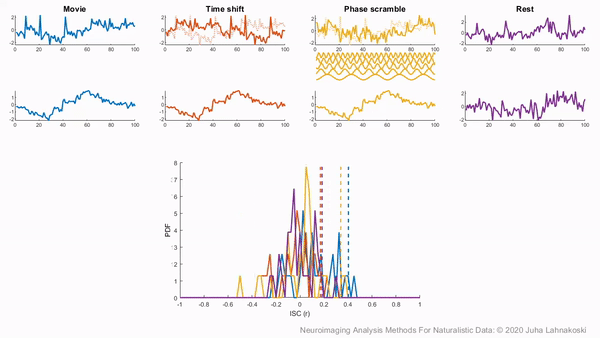The second approach employs a subject-wise bootstrap on the pairwise similarity matrices. Essentially, participants are randomly sampled with replacement and then a new similarity matrix is computed with these resampled participants. As a consequence of the resampling procedure, sometimes the same subjects are sampled multiple times, which introduces correlation values of 1 off the diagonal. Summarizing the ISC using the median can minimize the impact of these outliers. These values are then shifted by the real summary statistics to produce an approximately zero-centered distribution. Python implementations in Brainiak and nltools convert these values to NaNs by default so that they are not included in the overall ISC summary statistic. If you would like to learn more about resampling methods, we encourage you to read our brief introduction available on the dartbrains course.

## Getting Started¶

Before getting started with this tutorial, we need to make sure you have the necessary software installed and data downloaded.

### Software¶

This tutorial requires the following Python packages to be installed. See the Software Installation tutorial for more information.

• seaborn

• matplotlib

• numpy

• scipy

• pandas

• nltools

• nilearn

• sklearn

• networkx

Let’s now load the modules we will be using for this tutorial.

%matplotlib inline

import os
import glob
import numpy as np
from numpy.fft import fft, ifft, fftfreq
import pandas as pd
import matplotlib.pyplot as plt
from matplotlib import gridspec
from matplotlib.animation import FuncAnimation
import seaborn as sns
from nltools.stats import isc, isfc, isps, fdr, threshold, phase_randomize, circle_shift, _butter_bandpass_filter, _phase_mean_angle, _phase_vector_length
from nilearn.plotting import view_img_on_surf, view_img
from sklearn.metrics import pairwise_distances
from sklearn.utils import check_random_state
from scipy.stats import ttest_1samp
from scipy.signal import hilbert
import networkx as nx
from IPython.display import HTML

# import nest_asyncio
# nest_asyncio.apply()

/Users/lukechang/anaconda3/lib/python3.7/site-packages/matplotlib/image.py:13: RuntimeWarning: coroutine 'run_async_cmd' was never awaited
import PIL.PngImagePlugin
RuntimeWarning: Enable tracemalloc to get the object allocation traceback


### Data¶

This tutorial will be using the Sherlock dataset and will require downloading the Average ROI csv files.

We have already extracted the data for you to make this easier and have written out the average activity within each ROI into a separate csv file for each participant. If you would like to get practice doing this yourself, here is the code we used. Note that we are working with the hdf5 files as they load much faster than the nifti images, but either one will work for this example.

for scan in ['Part1', 'Part2']:
file_list = glob.glob(os.path.join(data_dir, 'fmriprep', '*', 'func', f'*crop*{scan}*hdf5'))
for f in file_list:
sub = os.path.basename(f).split('_')
print(sub)
data = Brain_Data(f)
pd.DataFrame(roi.T).to_csv(os.path.join(os.path.dirname(f), f"{sub}_{scan}_Average_ROI_n50.csv" ), index=False)


You will want to change data_dir to wherever you have installed the Sherlock datalad repository. We will initialize a datalad dataset instance and get the files we need for this tutorial. If you’ve already downloaded everything, you can skip this cell. See the Download Data Tutorial for more information about how to install and use datalad.

data_dir = '/Volumes/Engram/Data/Sherlock'

# If dataset hasn't been installed, clone from GIN repository
if not os.path.exists(data_dir):
dl.clone(source='https://gin.g-node.org/ljchang/Sherlock', path=data_dir)

# Initialize dataset
ds = dl.Dataset(data_dir)

# Get Cropped & Denoised CSV Files
result = ds.get(glob.glob(os.path.join(data_dir, 'fmriprep', '*', 'func', f'*Average_ROI*csv')))


## ISC Analysis Tutorial¶

Ok, now let’s see how ISC works with real data. In this tutorial, we will be computing ISC on average activity within 50 ROIs using data from the Sherlock dataset using the nltools Python toolbox. We also recommend checking out the Brainiak tutorial, and the Matlab ISC-Toolbox (Kauppi et al., 2014).

Ok, now let’s download the k=50 whole brain meta-analytic parcellation of the neurosynth database (de la Vega, 2016) from neurovault. Each ROI is indicated with a unique integer. We can expand this mask into 50 separate binary masks with the expand_mask function.

mask = Brain_Data('http://neurovault.org/media/images/2099/Neurosynth%20Parcellation_0.nii.gz')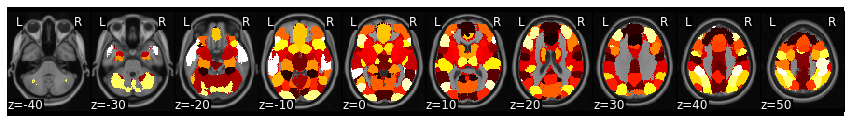Now, let’s load the csv files for each participant. Remember, Sherlock viewing was separated into two 25 minute runs. We will need to combine these separately for each participant. There are many different ways to do this step. In this example, we will be storing everything in a dictionary.

sub_list = [os.path.basename(x).split('_') for x in glob.glob(os.path.join(data_dir, 'fmriprep', '*', 'func', '*Part1*csv'))]
sub_list.sort()

sub_timeseries = {}
for sub in sub_list:
part1 = pd.read_csv(os.path.join(data_dir, 'fmriprep', sub, 'func', f'{sub}_Part1_Average_ROI_n50.csv'))
part2 = pd.read_csv(os.path.join(data_dir, 'fmriprep', sub, 'func', f'{sub}_Part2_Average_ROI_n50.csv'))
sub_data = part1.append(part2)
sub_data.reset_index(inplace=True, drop=True)
sub_timeseries[sub] = sub_data


Now, let’s pick a single ROI to demonstrate how to perform ISC analyses. We can pick any region, but let’s start with the vmPFC (roi=32), feel free to play with different regions as you work with the code.

We can plot the mask and create a new pandas DataFrame that has the average vmPFC activity for each participant.

roi = 32

def get_subject_roi(data, roi):
sub_rois = {}
for sub in data:
sub_rois[sub] = data[sub].iloc[:, roi]
return pd.DataFrame(sub_rois)

sub_rois = get_subject_roi(sub_timeseries, roi)

sub-01 sub-02 sub-03 sub-04 sub-05 sub-06 sub-07 sub-08 sub-09 sub-10 sub-11 sub-12 sub-13 sub-14 sub-15 sub-16
0 3.362605 -1.967253 -0.243505 2.527032 5.166227 -0.678549 2.199253 -1.646883e+00 0.421235 0.500547 0.361623 4.639737e+00 1.490442 1.806639 1.039467 3.483579e-13
1 0.995695 1.730923 1.552836 1.068784 4.066954 0.117737 3.184899 8.464993e-01 -0.118011 0.981400 -0.069505 2.522244e+00 1.145760 -0.582861 -0.420722 -1.237187e-13
2 2.084567 -1.940155 1.914897 1.103097 2.168681 0.030628 2.036096 1.782011e-01 0.984125 3.957482 -0.792416 1.326291e+00 0.472309 -3.066318 0.869296 -1.931528e-02
3 -0.217049 -0.636084 1.501459 -0.701397 1.704406 0.042397 2.353035 1.088203e+00 1.650786 3.687806 3.839885 2.105321e-02 -2.885314 -1.212683 1.213115 -1.460159e+00
4 -2.628723 1.650023 -1.196258 0.079026 1.297944 -0.743593 1.188282 3.375227e-13 1.515944 -0.709527 4.874887 2.279356e-13 -5.277045 0.232831 1.914874 1.745742e+00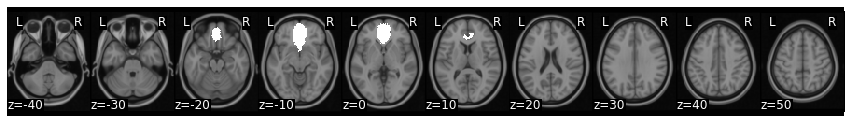### Circle Shift Randomization¶

To perform ISC we will be using the nltools.stats.isc function. There are three different methods implemented to perform hypothesis tests (i.e., circular shifting data, phase randomization, and subject-wise bootstrap). We will walk through an example of how to run each one.

The idea behind circular shifting the data is to generate random surrogate data that has the same autoregressive and temporal properties of the original data (Lancaster et al., 2018). This is fairly straightforward and involves randomly selecting a time point to become the new beginning of the timeseries and then concatenating the rest of the data at the end so that it has the same length as the original data. Of course, there will potentially be a sudden change in the data where the two parts were merged.

To demonstrate how this works and that the circular shifted data has the same spectral properties of the original data, we will plot one subject’s time series and shift it using the nltools.stats.circle_shift function. Next to both timeseries we plot the coefficients from a fast fourier transform.

sub = 'sub-02'
sampling_freq = .5

f,a = plt.subplots(nrows=2, ncols=2, figsize=(15, 5))
a[0,0].plot(sub_rois[sub], linewidth=2)
a[0,0].set_ylabel('Avg Activity', fontsize=16)
a[0,1].set_xlabel('Time (TR)', fontsize=18)
a[0,0].set_title('Observed Data', fontsize=16)

fft_data = fft(sub_rois[sub])
freq = fftfreq(len(fft_data), 1/sampling_freq)
n_freq = int(np.floor(len(fft_data)/2))
a[0,1].plot(freq[:n_freq], np.abs(fft_data)[:n_freq], linewidth=2)
a[0,1].set_xlabel('Frequency (Hz)', fontsize=18)
a[0,1].set_ylabel('Amplitude', fontsize=18)
a[0,1].set_title('Power Spectrum', fontsize=18)

circle_shift_data = circle_shift(sub_rois[sub])
a[1,0].plot(circle_shift_data, linewidth=2, color='red')
a[1,0].set_ylabel('Avg Activity', fontsize=16)
a[1,0].set_xlabel('Time (TR)', fontsize=16)
a[1,0].set_title('Circle Shifted Data', fontsize=16)

fft_circle = fft(circle_shift_data)
a[1,1].plot(freq[:n_freq], np.abs(fft_circle)[:n_freq], linewidth=2, color='red')
a[1,1].set_xlabel('Frequency (Hz)', fontsize=18)
a[1,1].set_ylabel('Amplitude', fontsize=18)
a[1,1].set_title('Circle Shifted Power Spectrum', fontsize=18)

plt.tight_layout()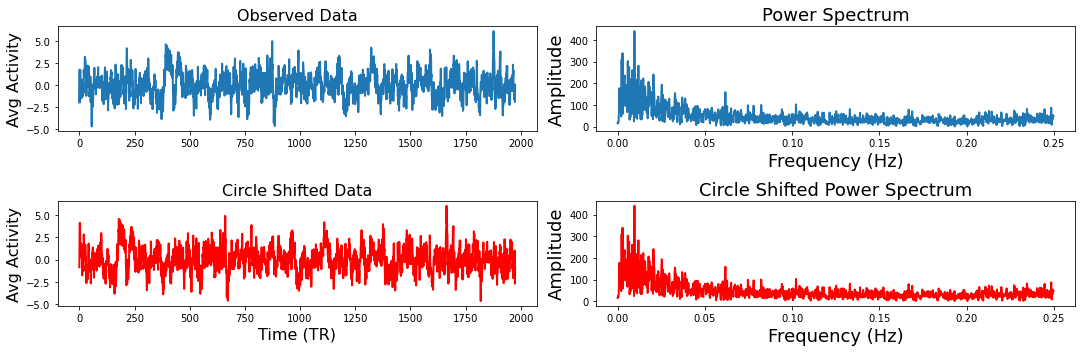Now that you understand how the circular shifting method works with a single time series, let’s compute the ISC of the Sherlock viewing data within the vmPFC roi with 5000 permutations. The function outputs a dictionary that contains the ISC values, the p-value, the 95% confidence intervals, and optionally returns the 5000 samples. All of the permutation and bootstraps are parallelized and will use as many cores as your computer has available.

stats_circle = isc(sub_rois, method='circle_shift', n_bootstraps=5000, return_bootstraps=True)

print(f"ISC: {stats_circle['isc']:.02}, p = {stats_circle['p']:.03}")

ISC: 0.074, p = 0.0002


### Phase Randomization¶

Next we will show how phase randomization works. This method projects the data into frequency space using a fast fourier transform and randomizes the phase and then projects the data back into the time domain (Theiler et al., 1992, Lancaster et al., 2018). Similar to the circular shifting method, this generates a random surrogate of the data, while maintaining a similar temporal and autoregressive structure as the original data. We will generate the same plots from the example above to illustrate how this works using the nltools.stats.phase_randomize function.

sub = 'sub-02'
sampling_freq = .5

f,a = plt.subplots(nrows=2, ncols=2, figsize=(15, 5))
a[0,0].plot(sub_rois[sub], linewidth=2)
a[0,0].set_ylabel('Avg Activity', fontsize=16)
a[0,1].set_xlabel('Time (TR)', fontsize=18)
a[0,0].set_title('Observed Data', fontsize=16)

fft_data = fft(sub_rois[sub])
freq = fftfreq(len(fft_data), 1/sampling_freq)
n_freq = int(np.floor(len(fft_data)/2))
a[0,1].plot(freq[:n_freq], np.abs(fft_data)[:n_freq], linewidth=2)
a[0,1].set_xlabel('Frequency (Hz)', fontsize=18)
a[0,1].set_ylabel('Amplitude', fontsize=18)
a[0,1].set_title('Power Spectrum', fontsize=18)

phase_random_data = phase_randomize(sub_rois[sub])
a[1,0].plot(phase_random_data, linewidth=2, color='red')
a[1,0].set_ylabel('Avg Activity', fontsize=16)
a[1,0].set_xlabel('Time (TR)', fontsize=16)
a[1,0].set_title('Phase Randomized Data', fontsize=16)

fft_phase = fft(phase_random_data)
a[1,1].plot(freq[:n_freq], np.abs(fft_phase)[:n_freq], linewidth=2, color='red')
a[1,1].set_xlabel('Frequency (Hz)', fontsize=18)
a[1,1].set_ylabel('Amplitude', fontsize=18)
a[1,1].set_title('Phase Randomized Power Spectrum', fontsize=18)

plt.tight_layout()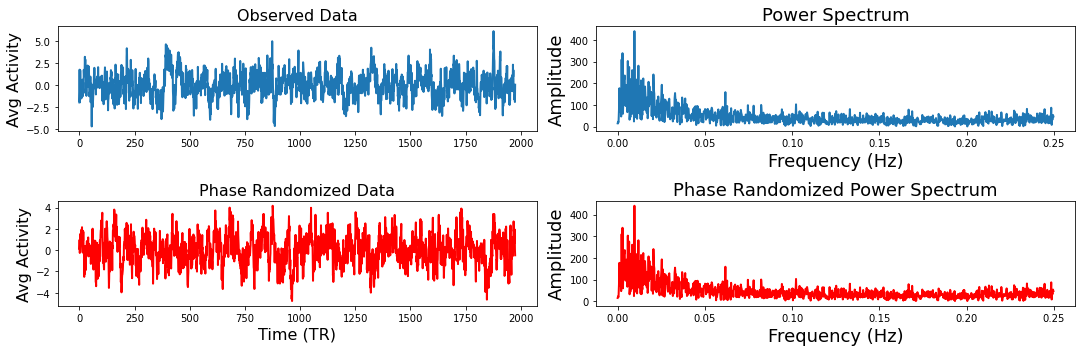stats_phase = isc(sub_rois, method='phase_randomize', n_bootstraps=5000, return_bootstraps=True)

print(f"ISC: {stats_phase['isc']:.02}, p = {stats_phase['p']:.03}")

ISC: 0.074, p = 0.0002


As you can see the ISC value is identical as the median of the pairwise correlations are identical. The p-values are also similar and likely reflect the limited precision of the possible p-values that can be computed using only 5,000 permutations. For greater precision, you will need to increase the number of permutations, but remember that this will also increase the computational time.

### Subject-wise Bootstrapping¶

The final approach we will illustrate is subject-wise bootstrapping of the pairwise similarity matrix. This approach is more conservative than the previously described methods and is almost an order of magnitude faster shuffling the similarity matrix rather than recomputing the pairwise similarity for the null distribution (Chen et al., 2016). Bootstrapping and permutation tests are different types of resampling statistics (see our resampling tutorial for a more in depth overview). Bootstrapping is typically used more for generating confidence intervals around an estimator, while permutation tests are used for performing hypothesis tests. However, p-values can also be computed using a bootstrap by subtracting the ISC from the null distribution and evaluating the percent of samples from the distribution that are smaller than the observed ISC (Hall et al., 1991).

Just like the examples above, we will illustrate what an example bootstrapped similarity matrix looks like. Notice how some subjects are repeatedly resampled, which means that there are multiple values of perfect correlations found off the diagonal. This should be accounted for by using the median summary statistic of the lower triangle. However, both Brainiak and nltools toolboxes convert these values to NaNs to minimize the impact of these outliers on the summary statistic.

def bootstrap_subject_matrix(similarity_matrix, random_state=None):
'''This function shuffles subjects within a similarity matrix based on recommendation by Chen et al., 2016'''

random_state = check_random_state(random_state)
n_sub = similarity_matrix.shape
bootstrap_subject = sorted(random_state.choice(np.arange(n_sub), size=n_sub, replace=True))
return similarity_matrix[bootstrap_subject, :][:, bootstrap_subject]

similarity = 1 - pairwise_distances(pd.DataFrame(sub_rois).T, metric='correlation')

f,a = plt.subplots(ncols=2, figsize=(12, 6), sharey=True)
sns.heatmap(similarity, square=True, cmap='RdBu_r', vmin=-1, vmax=1, xticklabels=False, yticklabels=False, ax=a)
a.set_ylabel('Subject', fontsize=18)
a.set_xlabel('Subject', fontsize=18)
a.set_title('Pairwise Similarity', fontsize=16)

sns.heatmap(bootstrap_subject_matrix(similarity), square=True, cmap='RdBu_r', vmin=-1, vmax=1, xticklabels=False, yticklabels=False, ax=a)
a.set_ylabel('Subject', fontsize=18)
a.set_xlabel('Subject', fontsize=18)
a.set_title('Bootstrapped Pairwise Similarity', fontsize=16)

Text(0.5, 1.0, 'Bootstrapped Pairwise Similarity')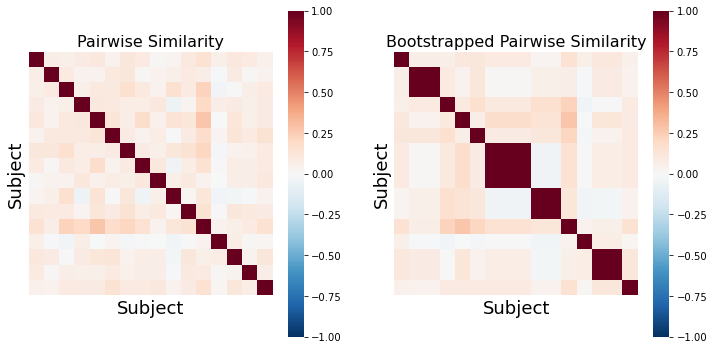stats_boot = isc(sub_rois, method='bootstrap', n_bootstraps=5000, return_bootstraps=True)

print(f"ISC: {stats_boot['isc']:.02}, p = {stats_boot['p']:.03}")

ISC: 0.074, p = 0.0002


The bootstrap procedure tends to run much faster than the permutation methods on our computer, which is one of the reasons that the authors of the toolbox recommend this approach, beyond it being more conservative (Chen et al., 2016).

### Null Distributions¶

Since each of our examples saved the null distribution, we can plot a histogram of the null distribution from each method including the confidence intervals. Notice that the circle shift and phase randomization methods produce highly similar null distributions and confidence intervals, while the bootstrap method has a wider and less symmetric distribution with the current number of iterations. However, the observed ISC of 0.074 (red line) exceeds all of the samples from the null distribution yielding a very small p-value. Notice that our observed null distributions using the surrogate data derived from the Sherlock data are very similar to the animation of null data presented above.

plt.figure(figsize=(12,6))
sns.distplot(stats_boot['null_distribution'] - stats_boot['isc'], kde=True, label='Bootstrap')
sns.distplot(stats_circle['null_distribution'], kde=True, label='Bootstrap')
sns.distplot(stats_phase['null_distribution'], kde=True, label='Bootstrap')
plt.ylabel('Frequency', fontsize=18)
plt.xlabel('ISC Values (correlation)', fontsize=18)
plt.title('ISC Null Distribution', fontsize=20)
plt.axvline(stats_boot['isc'], linestyle='-', color='red', linewidth=4)
plt.legend(['Observed ISC', 'Bootstrap Null','Circle Shift Null', 'Phase Randomize Null'], fontsize=18)

plt.axvline(stats_boot['ci'] - stats_boot['isc'], linestyle='--', color='blue')
plt.axvline(stats_boot['ci'] - stats_boot['isc'], linestyle='--', color='blue')

plt.axvline(stats_circle['ci'], linestyle='--', color='orange')
plt.axvline(stats_circle['ci'], linestyle='--', color='orange')

plt.axvline(stats_phase['ci'], linestyle='--', color='green')
plt.axvline(stats_phase['ci'], linestyle='--', color='green')

/Users/lukechang/anaconda3/lib/python3.7/site-packages/seaborn/distributions.py:2557: FutureWarning: distplot is a deprecated function and will be removed in a future version. Please adapt your code to use either displot (a figure-level function with similar flexibility) or histplot (an axes-level function for histograms).
warnings.warn(msg, FutureWarning)
/Users/lukechang/anaconda3/lib/python3.7/site-packages/seaborn/distributions.py:2557: FutureWarning: distplot is a deprecated function and will be removed in a future version. Please adapt your code to use either displot (a figure-level function with similar flexibility) or histplot (an axes-level function for histograms).
warnings.warn(msg, FutureWarning)
/Users/lukechang/anaconda3/lib/python3.7/site-packages/seaborn/distributions.py:2557: FutureWarning: distplot is a deprecated function and will be removed in a future version. Please adapt your code to use either displot (a figure-level function with similar flexibility) or histplot (an axes-level function for histograms).
warnings.warn(msg, FutureWarning)

<matplotlib.lines.Line2D at 0x7f8b50b81ad0>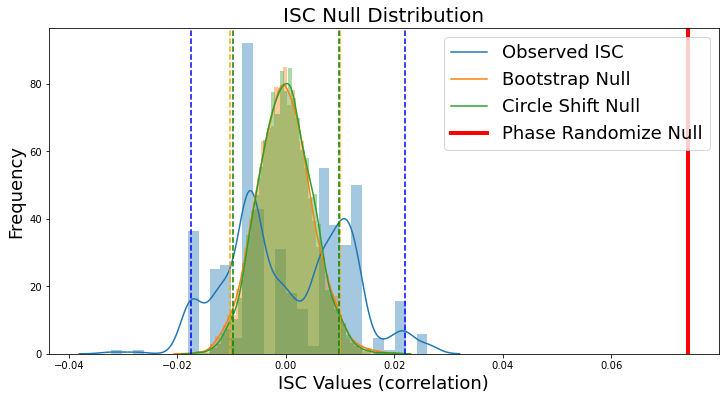### Whole brain ISC¶

Now, let’s calculate ISC looping over each of the 50 ROIs from the whole-brain meta-analytic parcellation (de la Vega, 2016). Here we loop over each ROI and grab the column from each subject’s dataframe. We then run ISC on the combined subject’s ROI timeseries using the median method and compute a hypothesis test using the subject-wise bootstrap method with 5000 samples (Chen et al., 2016). Finally, we convert each correlation and p-value from each region back into a Brain_Data instance.

isc_r, isc_p = {}, {}
for roi in range(50):
stats = isc(get_subject_roi(sub_timeseries, roi), n_bootstraps=5000, metric='median', method='bootstrap')
isc_r[roi], isc_p[roi] = stats['isc'], stats['p']


Now we can plot the ISC values to visualize which regions had a higher overall ISC.

isc_r_brain.plot(cmap='RdBu_r')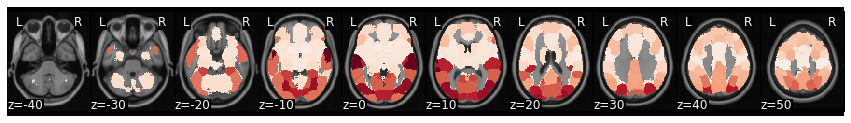view_img(isc_r_brain.to_nifti())


We can threshold using bonferroni correction (p < 0.001 for k=50 parcellation). Alternatively, we can threshold using false discovery rate, by setting thr=fdr(isc_p_brain.data). In this example, FDR is more conservative than bonferroni.

view_img_on_surf(threshold(isc_r_brain, isc_p_brain, thr=.001).to_nifti())